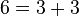# Direct product of Z8 and Z8

View a complete list of particular groups (this is a very huge list!)[SHOW MORE]

## Definition

This group can be defined as the external direct product of two copies of the cyclic group of order eight. In other words, it has the presentation:$G := \langle x,y \mid x^8 = y^8 = e, xy = yx \rangle$.

## As an abelian group of prime power order

This group is the abelian group of prime power order corresponding to the partition:$\! 6 = 3 + 3$

In other words, it is the group$\mathbb{Z}_{p^3} \times \mathbb{Z}_{p^3}$.

Value of prime number$p$ Corresponding group
generic prime direct product of cyclic group of prime-cube order and cyclic group of prime-cube order
3 direct product of Z27 and Z27
5 direct product of Z125 and Z125

## Arithmetic functions

Want to compare and contrast arithmetic function values with other groups of the same order? Check out groups of order 64#Arithmetic functions
Function Value Similar groups Explanation for function value
underlying prime of p-group 2
order (number of elements, equivalently, cardinality or size of underlying set) 64 groups with same order
prime-base logarithm of order 6 groups with same prime-base logarithm of order
max-length of a group 6 max-length of a group equals prime-base logarithm of order for group of prime power order
chief length 6 chief length equals prime-base logarithm of order for group of prime power order
composition length 6 composition length equals prime-base logarithm of order for group of prime power order
exponent of a group 8 groups with same order and exponent of a group | groups with same prime-base logarithm of order and exponent of a group | groups with same exponent of a group
prime-base logarithm of exponent 3 groups with same order and prime-base logarithm of exponent | groups with same prime-base logarithm of order and prime-base logarithm of exponent | groups with same prime-base logarithm of exponent
Frattini length 3 groups with same order and Frattini length | groups with same prime-base logarithm of order and Frattini length | groups with same Frattini length Frattini length equals prime-base logarithm of exponent for abelian group of prime power order
minimum size of generating set 2 groups with same order and minimum size of generating set | groups with same prime-base logarithm of order and minimum size of generating set | groups with same minimum size of generating set
subgroup rank of a group 2 groups with same order and subgroup rank of a group | groups with same prime-base logarithm of order and subgroup rank of a group | groups with same subgroup rank of a group same as minimum size of generating set since it is an abelian group of prime power order
rank of a p-group 2 groups with same order and rank of a p-group | groups with same prime-base logarithm of order and rank of a p-group | groups with same rank of a p-group same as minimum size of generating set since it is an abelian group of prime power order
normal rank of a p-group 2 groups with same order and normal rank of a p-group | groups with same prime-base logarithm of order and normal rank of a p-group | groups with same normal rank of a p-group same as minimum size of generating set since it is an abelian group of prime power order
characteristic rank of a p-group 2 groups with same order and characteristic rank of a p-group | groups with same prime-base logarithm of order and characteristic rank of a p-group | groups with same characteristic rank of a p-group same as minimum size of generating set since it is an abelian group of prime power order
nilpotency class 1 The group is a nontrivial abelian group
derived length 1 The group is a nontrivial abelian group
Fitting length 1 The group is a nontrivial abelian group

-

## Group properties

Property Satisfied? Explanation
cyclic group No
homocyclic group Yes
metacyclic group Yes
elementary abelian group No
abelian group Yes
group of prime power order Yes
nilpotent group Yes
solvable group Yes

## GAP implementation

### Group ID

This finite group has order 64 and has ID 2 among the groups of order 64 in GAP's SmallGroup library. For context, there are groups of order 64. It can thus be defined using GAP's SmallGroup function as:

SmallGroup(64,2)

For instance, we can use the following assignment in GAP to create the group and name it$G$:

gap> G := SmallGroup(64,2);

Conversely, to check whether a given group$G$ is in fact the group we want, we can use GAP's IdGroup function:

IdGroup(G) = [64,2]

or just do:

IdGroup(G)

to have GAP output the group ID, that we can then compare to what we want.

### Other descriptions

The group can be defined using the DirectProduct and CyclicGroup functions as:

DirectProduct(CyclicGroup(8),CyclicGroup(8))